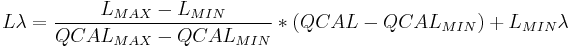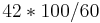# Difference between revisions of "Atmospheric correction"

Aim of this tutorial:

• Transforming a Landsat ETM+ image from DN values to radiance values
• Conducting an atmospheric correction of a Landsat image using the i.atcorr module in GRASS GIS and convert radiance to reflectance values

## Introduction

Standard steps for pre-processing satellite images are:

1. Converting Digital Numbers (DN) to Top-of-Atmosphere Radiances (ToAR)
• for Landsat imagery, apply the equation below or use the i.landsat.toar module
• DNs -- which are actually the pixel values -- are the result of the quantified amount of energy observed and measured at the sensor
• the i.landsat.toar module supports all Landsat sensors icluding MSS, TM and ETM+
2. Converting ToA Radiances to Top-of-Canopy Reflectances (ToCR) → use the i.atcorr module
• the conversion to Reflectances attempts to assess for and remove atmospheric effects, a process known as atmospheric correction
• the atmospheric correction step is quite a common topic of discussion in Remote Sensing. However, depending on the quality of the acquisition(s), it can be a complex process that does not always offer a great benefit, in terms of accuracy for subsequent processing steps (e.g. image segmentation and classification).

Remember to check if water areas are represented by values >0, since reflectance is always positive. If you encounter negative reflectance values, you have a "cornichon" (pickle in English ;-0). This means that the transformation equations used, do not correspond to the image processed.

## Requirements

After downloading of the North Carolina dataset, it must be unzipped into your GIS database. When starting GRASS GIS, select this GIS database, open the ‘nc_spm_08’ dataset as LOCATION, and ‘PERMANENT’ as MAPSET.

The NC data set mapset contains, amongst others, a Landsat ETM+ image of 24th of May 2002. Every pixel of this image contains a DN or grey value. In order to be able to make calculations with satellite imagery, or compare values amongst different sensors, these values have to be converted to radiances or reflectances. The formulas to do this conversion are described here for Landsat images (or use i.landsat.toar). The conversion from DN values to top-of-atmosphere radiances is done as follows:$L\lambda = \frac{L_{MAX}λ - L_{MIN}λ}{QCAL_{MAX} - QCAL_{MIN}} * (QCAL - QCAL_{MIN}) + L_{MIN}\lambda$

Where:

• - spectral Radiance at the sensor's aperture in watts/(meter squared * ster * μm), the apparent radiance as seen by the satellite sensor;
• QCAL - the quantized calibrated pixel value in DN;
• LMINλ - the spectral radiance that is scaled to QCALMIN in watts/(meter squared * ster * μm);
• LMAXλ - the spectral radiance that is scaled to QCALMAX in watts/(meter squared * ster * μm);
• QCALMIN - the minimum quantized calibrated pixel value (corresponding to LMINλ) in DN;
• QCALMAX - the maximum quantized calibrated pixel value (corresponding to LMAXλ) in DN=255.

LMINλ and LMAXλ are the radiances related to the minimal and maximal DN value, and are reported in the metadata file for each image, or in the table 1. High gain or low gain is also reported in the metadata file of each Landsat image. The minimal DN value (QCALMIN) is 1 for Landsat ETM+ images (1), and the maximal DN value (QCALMAX) is 255. QCAL is the DN value for every separate pixel in the Landsat image.

r.info lsat7_2002_xx


Under ‘comments’, the maximal and minimal radiance (LMAX and LMIN) for each band are given.

Power users may use this for nicely readable output (example for channel 1):

CHAN=1
r.info lsat7_2002_${CHAN}0 -h | tr '\n' ' ' | sed 's+ ++g' | tr ':' '\n' | grep "LMIN_BAND${CHAN}\|LMAX_BAND\${CHAN}"


Conversion to radiance (this calculation is done for band 1, for the other bands, the numbers in italics need to be replaced with their correct values):

g.region rast=lsat7_2002_10 -p
r.mapcalc "lsat7_2002_10_rad=((''191.6'' - (''-6.2'')) / (255.0 - 1.0)) * (lsat7_2002_10 - 1.0) + (''-6.2'')"


For faster computations, use an integer DEM:

 r.mapcalc "elev_int = round(elevation)"


Find mean elevation to initialize computations (needed in 'icnd' file below)

r.univar elev_int


## Estimating the overpass time from the sun position

The satellite overpass time can be estimated rather precisely from the sun position reported in metadata using r.sunmask. The metadata file for the following example contains: SUN_AZIMUTH = 120.8810347, SUN_ELEVATION = 64.7730999.

# iteratively change hour and minutes to obtain a good result, timezone needs to be correct of course
r.sunmask  -s elev=elevation out=dummy year=2002 month=5 day=24 hour=10 min=42 sec=7 timezone=-5


The above command reports:

Solar position: sun azimuth: 121.342461, sun angle above horz.(refraction corrected): 65.396652


The resulting overpass local time 10:42:07 corresponds to 15:42 in GMT which corresponds to 15.70 in decimal GMT hours (decimal minutes:$42 * 100 / 60$). This value is needed for the control file. For details, refer to Wikipedia's entry on Scientific decimal time.

## Atmospheric correction

This radiance image can be used for the atmospheric correction with the 6S algorithm. The algorithm will transform the top-of-the-atmosphere radiance values to top-of-canopy reflectance values using predefined information on the aerosol content and atmospheric composition of the time the image was taken. What follows describes the method to use this algorithm in GRASS GIS. Again, only the calculations for band 1 are shown, and the numbers to be changed for the other bands are indicated in red. The 'icnd_lsat1.txt' control file consists of the following parameters, and is written with a text editor:

8                          # indicates that it is an ETM+ image
05 24 15.67 -78.691 35.749 # image taken on the 24th of May, at 15:42 GMT in decimals; the center of the image lies at 78.691°W and 35.749°N
2                          # the midlatitude summer atmospheric model
1                          # the continental aerosol model
50                         # the visibility for the aerosol model [km]
-0.110                     # the terrain lies 110 meters above sea level [km] * -1
-1000                      # image taken of a satellite sensor (1000)
61                         # spectral band, here 1: blue


TODO: understand if the MODIS Aerosol Product (MOD04) could be useful to estimate the visibility for the aerosol model.

This file is then used in the i.atcorr module:

i.atcorr -a -o iimg=lsat7_2002_10_rad ialt=elev_int icnd=icdn_lsat1.txt oimg=lsat7_2002_10_atcorr


Where:

• -a refers to a Landsat image taken after July 2000
• -o activates the cache acceleration
• iimg is the image to be corrected
• ialt is the altitude map which overrides the initialization value of 110 meters
• icnd is the path to the icnd.txt file
• oimg is the name of the output image

Detailed information can be found in i.atcorr's manual.